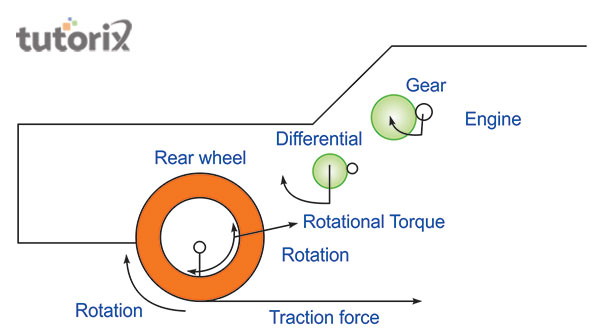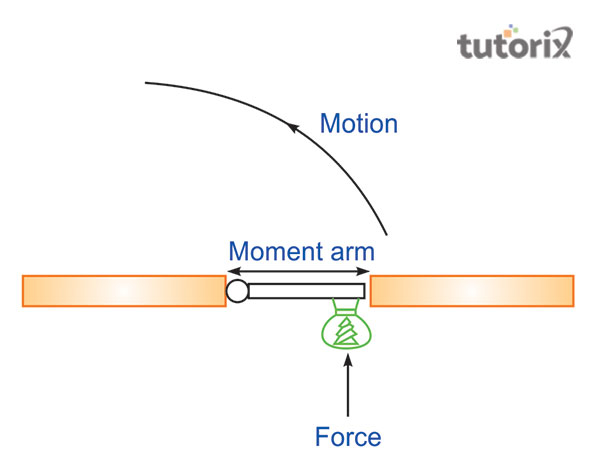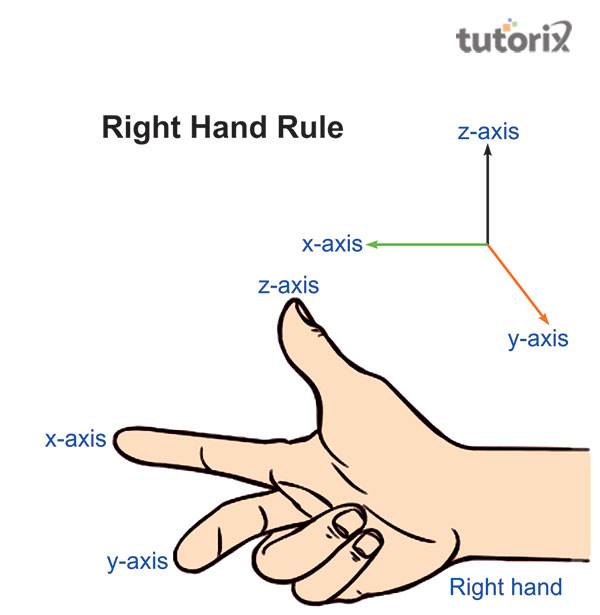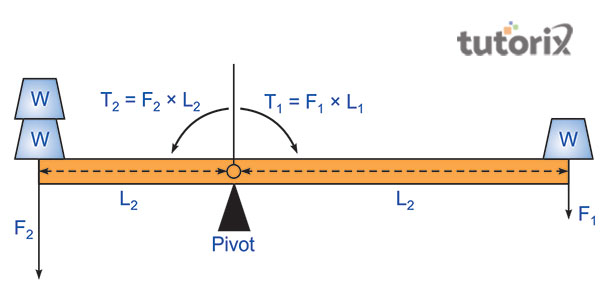# Torque

Torque is a wider topic in physics that has different applications despite its concerns with automobiles. The rotational axis is defined as the point at which the material is rotating. Consider an instance of opening a locked door, where the torque is put over three times. The key is turned to unlock, the doorknob is turned and the door is pushed to get open. Torque is referred to as the product multiplication of a radius vector which is represented by the symbol T.

Torque is usually considered when a car's speed, force or power is comprehended. In the case of the seesaw, the arm moment of a heavy person is small in comparison to that of a lighter person. The heavy weight helps in minimising Torque by sitting nearer to the pivot. The torque is decreased permitting lighter weight to pull bigger material because the arm of level is smaller.

## What is Torque?

Torque is also illustrated as the force moment which has the tendency to move the object to which it has been applied. In other words, the turning impact of the force around the rotational axis is defined as torque or moment of force (Zhu, Ralph & Buhrman, 2021). The SI unit of torque is said to be Nm. Torque is also known as the rotational equivalent of a linear force.Figure 1: Torque in automobiles

Torque is discussed utilising a variety of terminologies including a moment of force and moment. The twisting force that helps in making the motion is demonstrated as the torque. Torque is basically a term that is utilised to illustrate the power of a car (Andriani et al. 2020). In daily life, it is the force that is exerted by pistons on the crankshaft which causes it and the wheels to shift around in a car.

## Types of torque

Torque has been classified into two types which include Dynamic and static torque.

• Static torque − It is defined as the torque that does not occur as angular acceleration. The static force is put on when an individual is closing the door because it does not move until the force is applied.Figure 2: Torque

• Dynamic torque − The driving shaft of acceleration of a racing car from the initiation line helps in carrying the dynamic torque. It is because it must create wheels’ angular acceleration if the vehicle is completely accelerating along the circuit.

## The distinction between Torque and force

Features Torque Force
Explanation When the force is put on a particular object it helps it to move around an axis. An action type that let the body transform into motion
Acceleration type Angular acceleration Linear acceleration most of the time
Equation T = F x r x sin(θ) F = ma
Metric Measurement unit Nm (Newton-metre) Newton
English measurement unit Foot pound Pound

Table 1: Distinction between Torque and force

## Determination of Torque by thumb rule

The right-hand thumb rule is utilised in identifying the torque vector's direction. The points of torque vector in the thumb direction if warped hand around the rotational axis with the fingers pointed in the direction of the fore.

• The right hand is turned in the position vector's direction (r and d) then the fingers are in force direction and the thumb is in torque direction.
• The thumb direction is altered when either the F direction or r direction is changed. Torque direction remains unaltered if both directions are altered.Figure 3: Right-hand thumb rule

• The force may not impact the rotational axis if the vector of force is around 0° or 180° (Kawaharazuka et al. 2019). It might get away or towards the rotational axis because it is in a similar direction. In this situation, the value of torque is zero.
• The most impactful vector of force for creating torque that is perpendicular to the situation is θ = 90°.

## Torque: Application

Application of Torque happening all around and some major applications are listed below −

• Wrench − A pulling force around the axis of rotation of the nut is applied when the wrench is utilised to loosen or tighten the nut around a bolt. It helps in permitting the torque to rotate or kick in (Li et al. 2019). The addition of overall forces must be considered and multiplied with direction from the point of application of force to the pivot point to obtain torque amount since multiple forces are performed simultaneouslyFigure 4: Torque (moment)

In equilibrium, T1 = T2,

F1 x L1 = F2 x L2 and Torque helps in equalising the force times distance, T = F x L.

• Steering wheel − A car's steering wheel is often smaller as compared to an 18-wheeler because turning an 18-wheeler needs huge force than doing for a vehicle (Huang et al. 2021). The force amount needed for the application of torque minimises making it feasible for the truck driver to move the steering wheel by maximising the steering wheel's radius from a pivot point.

## Conclusion

Torque is an extremely crucial physics concept that assists in understanding how things are going around. Torque is not utilised in cars only but is applied in myriad from anything as feasible as seesaws to complicated machinery and more. Torque has the tendency to impress the customers in commercials and advertising and lure them into trusting that large is always the better. An online torque converter is also there which assists in comprehending the concept more in detail and permits to see the torque value in distinct measurement systems.

## FAQs

Q1. Why do cars get faster?

The revolution power and torque product per minute is horsepower. The faster, the crankshaft rotates with a similar force amount, the more power an engine creates. The car with huge horsepower as compared to torque is rapid as it gives speed and acceleration.

Q2. What is the formula of Torque?

Torque is represented as T= F x r x sin (θ). Based on this formula, F is the linear force, r is the distance between the rotational axis and the point of application of force and θ denotes the angle between r and F.

Q3. Why is torque not energy?

Torque is not energy because it is a vector quantity. It occurs to have similar units.

Q4. Where does torque play a significant role?

Torque plays a significant role in different phases of daily life. Playground seesaws, opening a bottle of coke, steering wheels, and car engines are the instances of torque in action.